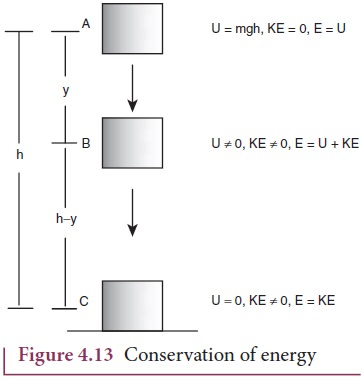# Principle of Conservation of Energy

• The energy is a conserved quantity. There won’t be any change in the energy of an isolated system.
• The energy can neither be created nor can it be destroyed. It can only be changed from its one form to another form.
• For example: if we throw a stone upward with certain velocity, initially, it has maximum velocity and hence maximum kinetic energy.
• As it moves up, its velocity goes on At a certain height, its velocity becomes zero, and hence its kinetic energy becomes zero.
• At the same time, the stone gains height as it moves up.
• As the stone gains height, its potential energy increases. Hence, it is clear that when a stone is thrown up, there is continuous decrease in kinetic energy and increase in the potential energy.
• However, the total energy of the stone remains the same. This is the principle of conservation of energy.
• According to principle of conservation of energy, the energy can neither be created nor be destroyed but can be changed from one form to another form.Image Source: nuclearpower                                             Image Source: Braincart

Energy conservation in free fall

Consider a body of mass m is initially at a point A which is at a height h from the ground as in the given figure. Let the body fall freely under gravity so that the acceleration of the body is g– acceleration due to gravity. After a certain time, the body reaches a point B which is at height (h-x) from the ground.As the body falls down, its velocity increases. Finally, the body strikes the ground C with velocity v.

For the point A

When the body is at A, velocity (u) = 0 and the height from the ground (h) = h.

Hence, the kinetic energy of the body   (K.E)a = ½ mu2 = 0

And the potential energy of the body is (PE)a = mgh

The total mechanical energy of body at point A is given by

Ea = (K.E)a + (P.E)a = 0 + mgh = mgh

∴ Ea = mgh …………………………………………………………. (i)

For the point B

When the body is at point B on its way down, we have initial velocity (u) = 0

Final velocity (v) = vb

Distance travelled = AB=AC – BC = h – (h-x) = x

We have, v2 = u2 + 2gh (formula)

Or, vb2 = 0 + 2gx = 2gx

∴ vb2 = 2gx

Now, the kinetic energy of the body at point B is

(K.E)b = ½ mvb2 = ½ m(2gx) = mgx

Also, the potential energy of the body at point B is

(P.E)b = mgh = mg(h-x)

Therefore, the total mechanical energy of the body when it is at the point B is given by

Eb = (K.E)b + (P.E)b = mgx + mg(h-x) = mgx + mgh – mgx = mgh

∴ Eb= mgh…………………………………………………………… (ii)

For the point C

When the body is at point C, we have, for the motion of the body

Initial velocity (u) = 0

Final velocity (v) = vc

Distance travelled = h

We have, v2= u2 + 2gh

or, vc2 = 0 + 2gh =2gh

∴vc2 = 2gh

Hence, when the body is at point C, the kinetic energy is given by,

(K.E)c = ½ mvc2 = ½ m(2gh) = mgh

Similarly, the potential energy of the body at the point C is given by,

(P.E)c = mg(height of point C) = mg × 0 = 0

Hence, the total mechanical energy of the body when it is at the point C is given by,

Ec = (K.E)c + (P.E)c = mgh + 0 = mgh

∴ Ec = mgh………………………………………………………… (iii)

From equation (i), (ii) and (iii), it is seen that the total mechanical energy of a freely falling body when it is at point A is equal to the energy when it is at B or C.

This implies that the total mechanical energy of a freely falling body is conserved.

In other words, the principle of conservation of energy holds true in this case of a freely falling body.

If a graph is plotted between the total mechanical energy of a freely falling body and its height from the ground, a curve is obtained as in figure below.References: